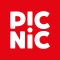# PrimitiveRules

SUGGESTION

Simplification

Suppression

Disable all rules by adding `-XepOpt:Refaster:NamePattern=^(?!(PrimitiveRules.*))` as compiler argument.

## LessThan

SUGGESTION

Simplification

Suppression

Suppress false positives by adding the suppression annotation `@SuppressWarnings("LessThan")` to the enclosing element.

Disable this rule by adding `-XepOpt:Refaster:NamePattern=^(?!(PrimitiveRules\.LessThan))` as compiler argument.

### Samples

Shows the difference in example code before and after the Refaster rule is applied.

`````` ImmutableSet<Boolean> testLessThan() {
return ImmutableSet.of(
-        !((byte) 3 >= (byte) 4),
-        !((char) 3 >= (char) 4),
-        !((short) 3 >= (short) 4),
-        !(3 >= 4),
-        !(3L >= 4L),
-        !(3F >= 4F),
-        !(3.0 >= 4.0));
+        (byte) 3 < (byte) 4,
+        (char) 3 < (char) 4,
+        (short) 3 < (short) 4,
+        3 < 4,
+        3L < 4L,
+        3F < 4F,
+        3.0 < 4.0);
}
``````

## LessThanOrEqualTo

SUGGESTION

Simplification

Suppression

Suppress false positives by adding the suppression annotation `@SuppressWarnings("LessThanOrEqualTo")` to the enclosing element.

Disable this rule by adding `-XepOpt:Refaster:NamePattern=^(?!(PrimitiveRules\.LessThanOrEqualTo))` as compiler argument.

### Samples

Shows the difference in example code before and after the Refaster rule is applied.

`````` ImmutableSet<Boolean> testLessThanOrEqualTo() {
return ImmutableSet.of(
-        !((byte) 3 > (byte) 4),
-        !((char) 3 > (char) 4),
-        !((short) 3 > (short) 4),
-        !(3 > 4),
-        !(3L > 4L),
-        !(3F > 4F),
-        !(3.0 > 4.0));
+        (byte) 3 <= (byte) 4,
+        (char) 3 <= (char) 4,
+        (short) 3 <= (short) 4,
+        3 <= 4,
+        3L <= 4L,
+        3F <= 4F,
+        3.0 <= 4.0);
}
``````

## GreaterThan

SUGGESTION

Simplification

Suppression

Suppress false positives by adding the suppression annotation `@SuppressWarnings("GreaterThan")` to the enclosing element.

Disable this rule by adding `-XepOpt:Refaster:NamePattern=^(?!(PrimitiveRules\.GreaterThan))` as compiler argument.

### Samples

Shows the difference in example code before and after the Refaster rule is applied.

`````` ImmutableSet<Boolean> testGreaterThan() {
return ImmutableSet.of(
-        !((byte) 3 <= (byte) 4),
-        !((char) 3 <= (char) 4),
-        !((short) 3 <= (short) 4),
-        !(3 <= 4),
-        !(3L <= 4L),
-        !(3F <= 4F),
-        !(3.0 <= 4.0));
+        (byte) 3 > (byte) 4,
+        (char) 3 > (char) 4,
+        (short) 3 > (short) 4,
+        3 > 4,
+        3L > 4L,
+        3F > 4F,
+        3.0 > 4.0);
}
``````

## GreaterThanOrEqualTo

SUGGESTION

Simplification

Suppression

Suppress false positives by adding the suppression annotation `@SuppressWarnings("GreaterThanOrEqualTo")` to the enclosing element.

Disable this rule by adding `-XepOpt:Refaster:NamePattern=^(?!(PrimitiveRules\.GreaterThanOrEqualTo))` as compiler argument.

### Samples

Shows the difference in example code before and after the Refaster rule is applied.

`````` ImmutableSet<Boolean> testGreaterThanOrEqualTo() {
return ImmutableSet.of(
-        !((byte) 3 < (byte) 4),
-        !((char) 3 < (char) 4),
-        !((short) 3 < (short) 4),
-        !(3 < 4),
-        !(3L < 4L),
-        !(3F < 4F),
-        !(3.0 < 4.0));
+        (byte) 3 >= (byte) 4,
+        (char) 3 >= (char) 4,
+        (short) 3 >= (short) 4,
+        3 >= 4,
+        3L >= 4L,
+        3F >= 4F,
+        3.0 >= 4.0);
}
``````

## LongToIntExact

SUGGESTION

Simplification

Suppression

Suppress false positives by adding the suppression annotation `@SuppressWarnings("LongToIntExact")` to the enclosing element.

Disable this rule by adding `-XepOpt:Refaster:NamePattern=^(?!(PrimitiveRules\.LongToIntExact))` as compiler argument.

### Samples

Shows the difference in example code before and after the Refaster rule is applied.

`````` int testLongToIntExact() {
-    return Ints.checkedCast(Long.MAX_VALUE);
+    return Math.toIntExact(Long.MAX_VALUE);
}
``````